Courses

# Test: Pressure & Its Measurement - 1

## 10 Questions MCQ Test Fluid Mechanics | Test: Pressure & Its Measurement - 1

Description
This mock test of Test: Pressure & Its Measurement - 1 for Mechanical Engineering helps you for every Mechanical Engineering entrance exam. This contains 10 Multiple Choice Questions for Mechanical Engineering Test: Pressure & Its Measurement - 1 (mcq) to study with solutions a complete question bank. The solved questions answers in this Test: Pressure & Its Measurement - 1 quiz give you a good mix of easy questions and tough questions. Mechanical Engineering students definitely take this Test: Pressure & Its Measurement - 1 exercise for a better result in the exam. You can find other Test: Pressure & Its Measurement - 1 extra questions, long questions & short questions for Mechanical Engineering on EduRev as well by searching above.
QUESTION: 1

### A hydraulic press has a ram of 30 cm diameter and a plunger of 4.5 cm diameter. What is the weight lifted by the hydraulic press when the force applied at the plunger is 600 N?

Solution: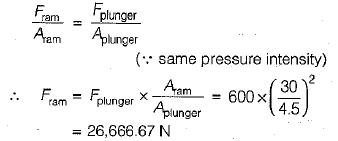QUESTION: 2

### An open tank contains water upto a depth of 2m and above it an oil of specific gravity 0.9 for a depth of 1m. The pressure intensity at the bottom of tank is  [Take g - 9.81 m/s2]

Solution: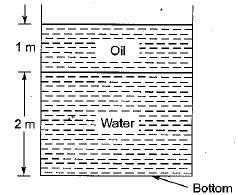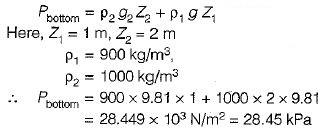QUESTION: 3

### Fluid statics deals with

Solution:
QUESTION: 4

Pascal’s law states that pressure at a point is equal in all directions

Solution:
QUESTION: 5

Gauge pressure at a point is equal to

Solution: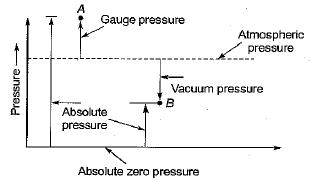∴ Gauge pressure = Absolute minus atmospheric pressure

QUESTION: 6

For a fluid at rest

Solution:
QUESTION: 7

Select the correct statement:

Solution:
QUESTION: 8

For a real fluid moving with uniform velocity, the pressure

Solution:

(d) In case of a real fluid moving with uniform velocity, the velocity head and pressure head are dependent on each other and their total sum remains constant. The pressure is thus independent of both depth and orientation, but in case of fluids under static condition, the pressure would depend on depth.

QUESTION: 9

If a Mohr circle is drawn for a fluid element inside a fluid body at rest, it would be

Solution: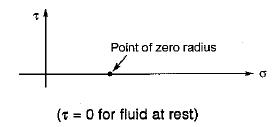QUESTION: 10

Bourdon gauage measures

Solution: